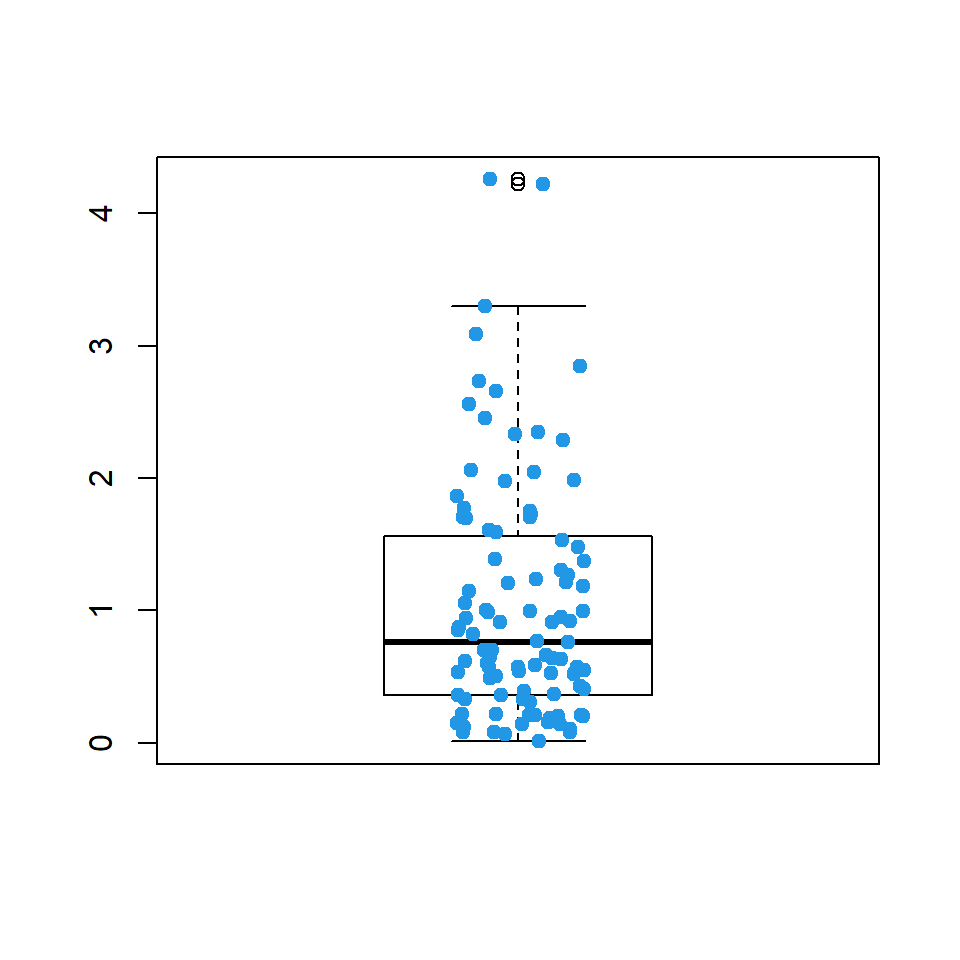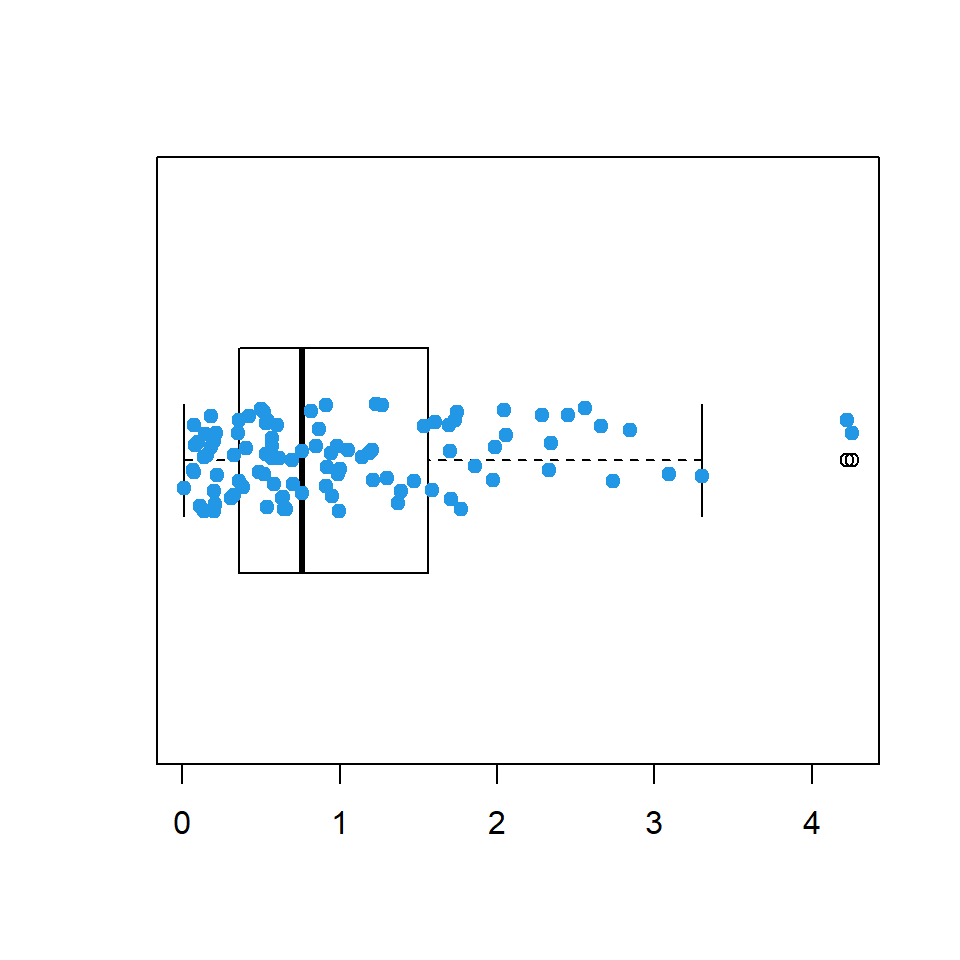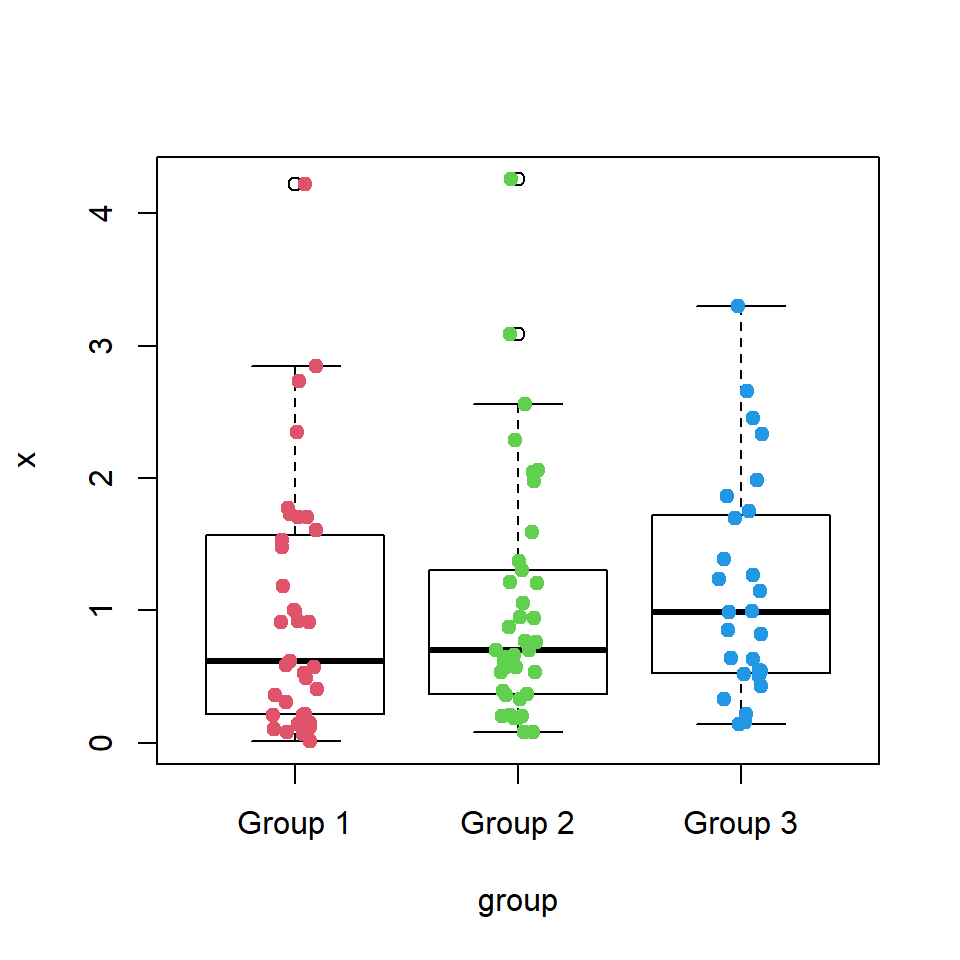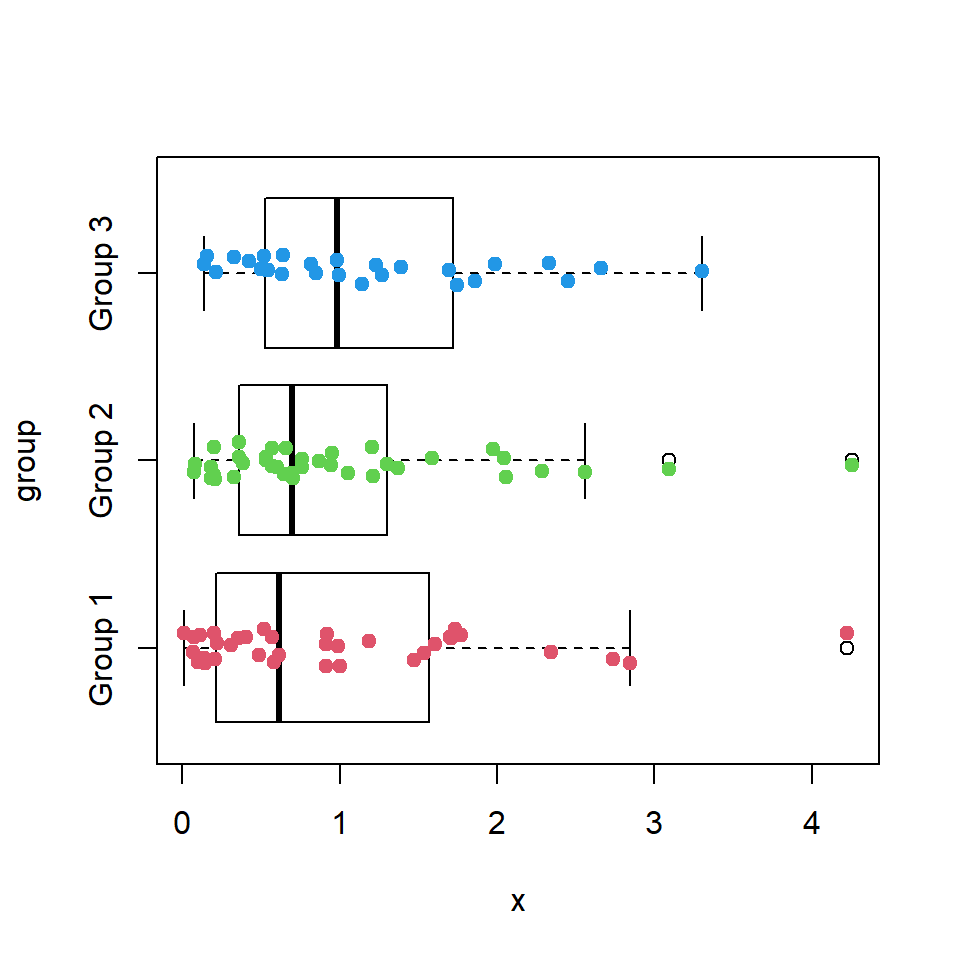# Adding points to box plots in R

## Sample data

Consider the following data set for this tutorial, where the variable `x` is a numeric variable drawn from an exponential distribution and `group` is a categorical variable with groups.

``````# Sample dataset
set.seed(3)
df <- data.frame(x = rexp(100),
group = sample(paste("Group", 1:3),
size = 100,
replace = TRUE))``````

## Single box plot with points

Adding points (strip charts) to a base R box plot can be achieved making use of the `stripchart` function. You need to pass the data you used to create your box plot, set the `"jitter"` method to add random noise over the data points, avoiding overplotting, set the desired aesthetics arguments such as `pch` or `col` and `add = TRUE` so the points are added over the previous plot. Recall that box plots are vertical by default, so you will need to set `vertical = TRUE`.

Vertical box plot with points

``````# Vertical box plot
boxplot(df\$x, col = "white")

# Points
stripchart(df\$x,              # Data
method = "jitter", # Random noise
pch = 19,          # Pch symbols
col = 4,           # Color of the symbol
vertical = TRUE,   # Vertical modeHorizontal box plot with points

``````# Horizontal box plot
boxplot(df\$x, col = "white", horizontal = TRUE)

# Points
stripchart(df\$x,              # Data
method = "jitter", # Random noise
pch = 19,          # Pch symbol
col = 4,           # Color of the symbol## Box plot by group with points

In order to add the points when working with a box plot by group you need to pass the same formula you used to create the box plot to the `stripchart` function. Recall that box plots are vertical by default, but stripcharts are not.Vertical box plot by group with points

``````# Vertical box plot by group
boxplot(x ~ group, data = df, col = "white")

# Points
stripchart(x ~ group,
data = df,
method = "jitter",
pch = 19,
col = 2:4,
vertical = TRUE,Horizontal box plot by group with points

``````# Horizontal box plot by group
boxplot(x ~ group, data = df, col = "white",
horizontal = TRUE)

# Points
stripchart(x ~ group,
data = df,
method = "jitter",
pch = 19,
col = 2:4,